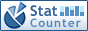Home PC Games Linux Windows Database Network Programming Server Mobile
 Home \ Programming \ List Leaves - Zabbix Agent (Server) - C ++ 11 feature: auto keyword (Programming) - Chrome plug-in management, online-offline installation, part of the plug presentations (Linux) - Python system default encoding (Programming) - How to write a new Git protocol (Linux) - 20+ Best Practices article MySQL Performance Optimization (Database) - how to quickly find a file on Linux system (Linux) - Unsafe reboot start (Linux) - How to install Git on CentOS 7 (Linux) - Linux operating system Start Tutorial: Xmanager Remote Access Linux graphical interface (Linux) - Windows environment Android Studio v1.0 Installation Guide (Linux) - Docker data container protection the pros and cons (Server) - Oracle database, some basic grammatical structures (Database) - Oracle Linux 5.9 configuration Xmanager (Linux) - Install and use automated tools Ansible in CentOS 7 (Linux) - Partition contrast manifestations under Windows and Linux (Linux) - MySQL Statistics (Database) - Common Linux system performance monitoring command (Linux) - Ubuntu 14.04 installed NVIDIA graphics driver (Linux) - AngularJS notes --- Scope and controller (Programming) List Leaves Add Date : 2017-04-13 Given a tree, you are supposed to list all the leaves in the order of top down, and left to right. Input Specification: Each input file contains one test case For each case, the first line gives a positive integer N (<= 10) which is the total number of nodes in the tree -. And hence the nodes are numbered from 0 to N-1. . Then N lines follow, each corresponds to a node, and gives the indices of the left and right children of the node If the child does not exist, a "-" will be put at the position Any pair of children are separated by. a space. Output Specification: For each test case, print in one line all the leaves' indices in the order of top down, and left to right. There must be exactly one space between any adjacent numbers, and no extra space at the end of the line. Sample Input: 8 1 - - - 0 - 27 - - - - 5 - 46 Sample Output: 415 Title to the effect: By entering the number of nodes and the left son and right son of each node, leaf nodes are printed upside down. Key topics: To understand the first few lines of the input node is to represent the value of a few. Such as sample input in the 0th row 1 - Represents the value of left child node 0 is 1, which points to the first line, the right child is empty (-1) Code is as follows: #include #include #define N 10 typedef struct Node {     int data, left, right; } TreeNode; TreeNode node [N]; TreeNode Queue [N]; // array queue int first = -1, last = -1; void Push (TreeNode tn); TreeNode Pop (); void printLeaves (int root, int n); int charToInt (char ch); int main () {     int n;     bool isRoot [N];     int root;     scanf ( "% d \ n", & n);     for (int i = 0; i         isRoot [i] = 1;     for (int i = 0; i     {         char cLeft, cRight;         scanf ( "% c% c", & cLeft, & cRight);         getchar (); // read carriage buffer zone         node [i] .left = charToInt (cLeft);         node [i] .right = charToInt (cRight);         node [i] .data = i;         // Node left child and a right child is certainly not the root         if (node [i] .left! = -1)             isRoot [node [i] .left] = 0;         if (node [i] .right! = -1)             isRoot [node [i] .right] = 0;     }     // Find the root     for (int i = 0; i     {         if (isRoot [i])         {             root = i;             break;         }     }     printLeaves (root, n);     return 0; } void Push (TreeNode treeNode) {     Queue [++ last] = treeNode; } TreeNode Pop () {     return Queue [++ first]; } // Node tree traversal sequence and print out a leaf node: queue implementation void printLeaves (int root, int n) {     int leaves [N];     int k = 0;     Push (node [root]);     for (int i = 0; i     {         TreeNode tn = Pop ();         // The left child and right child does not exist, it will save the value of the leaf node to the array, formatted for easy printing         if (tn.left == -1 && tn.right == -1)             leaves [k ++] = tn.data;         if (tn.left! = -1)             Push (node [tn.left]);         if (tn.right! = -1)             Push (node [tn.right]);     }     for (int i = 0; i         printf ( "% d", leaves [i]);     printf ( "% d \ n", leaves [k-1]); } int charToInt (char ch) {     if (isdigit (ch))         return ch - '0';     else         return -1; } More:
 CopyRight 2002-2022 newfreesoft.com, All Rights Reserved.